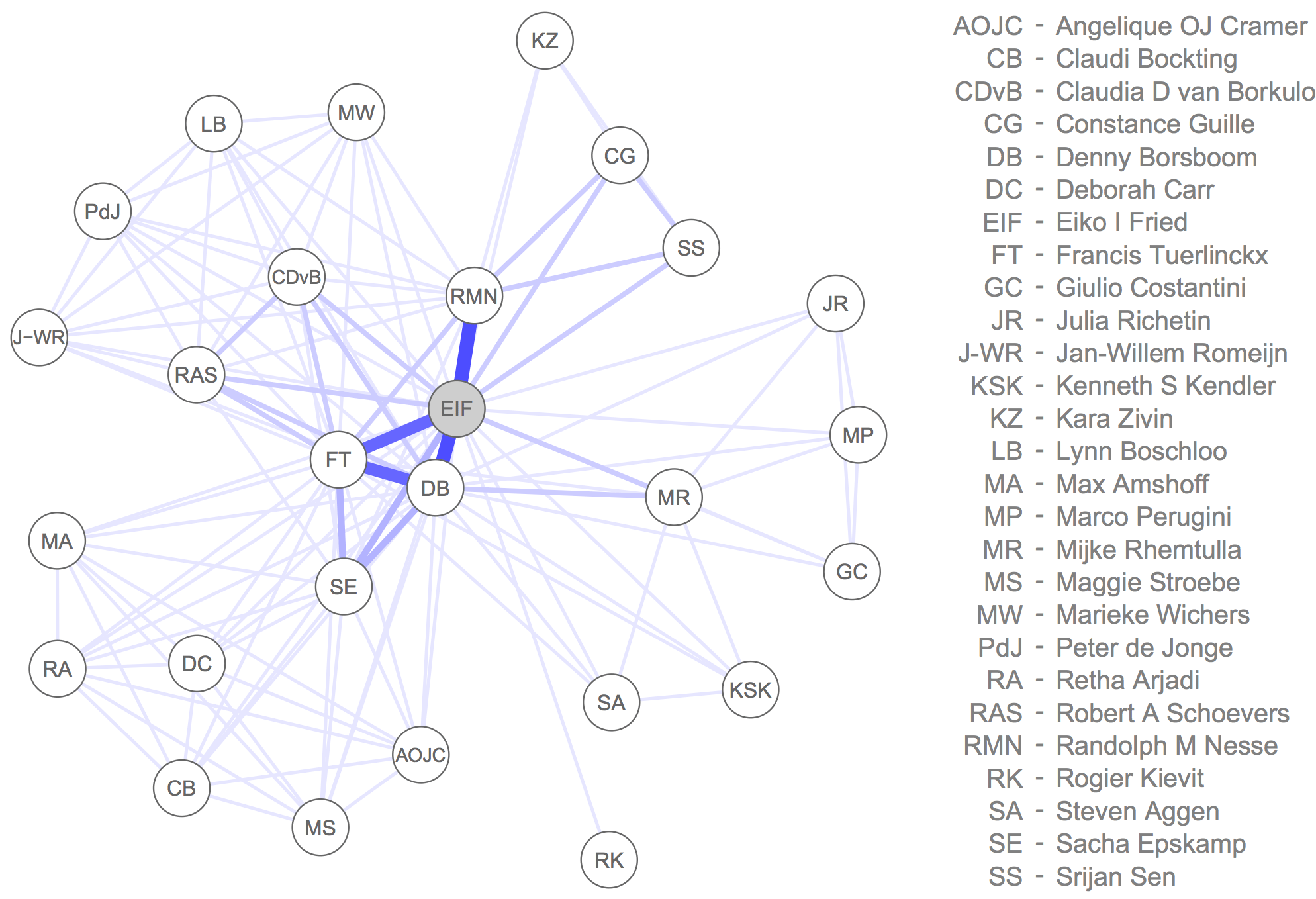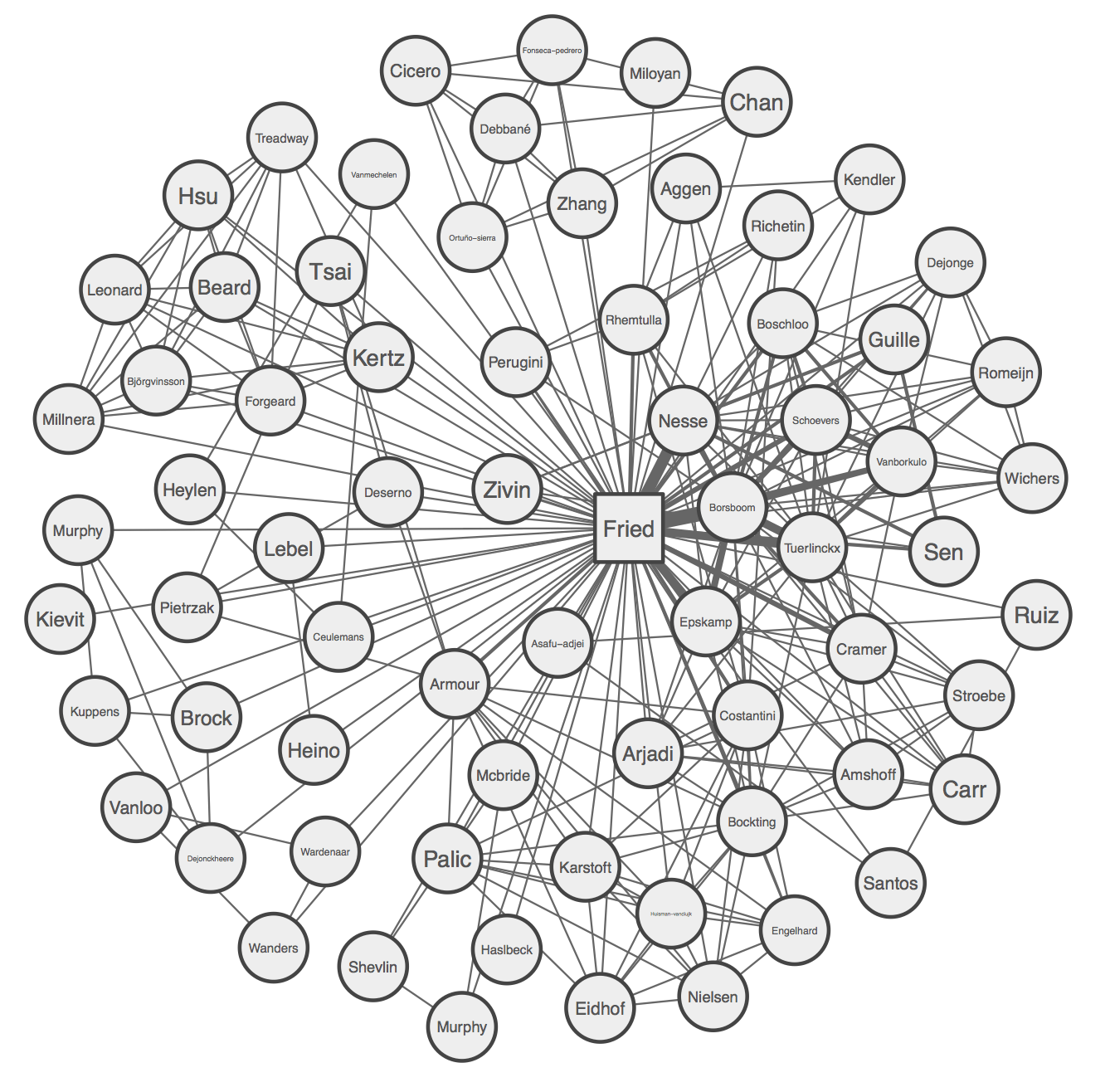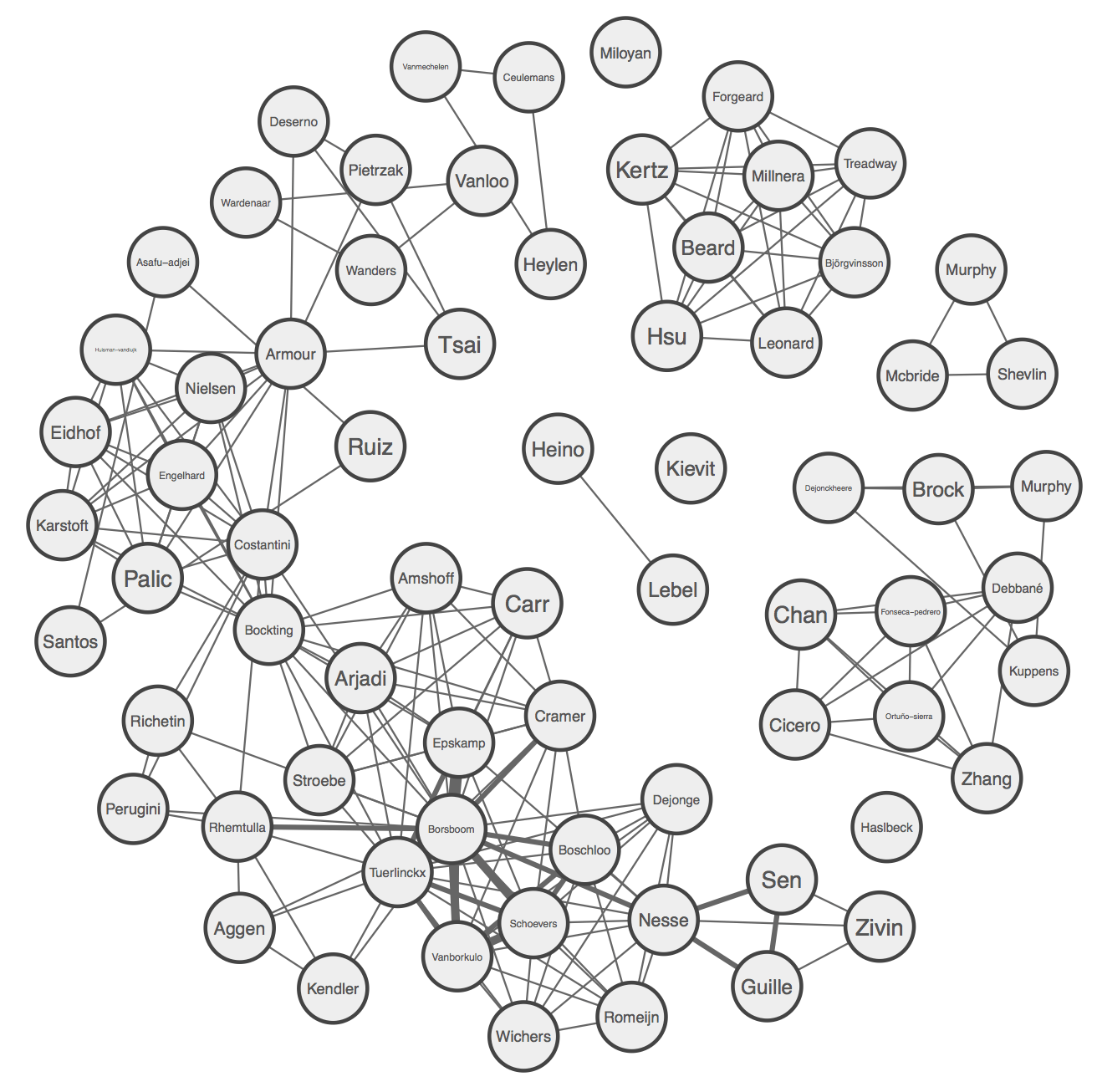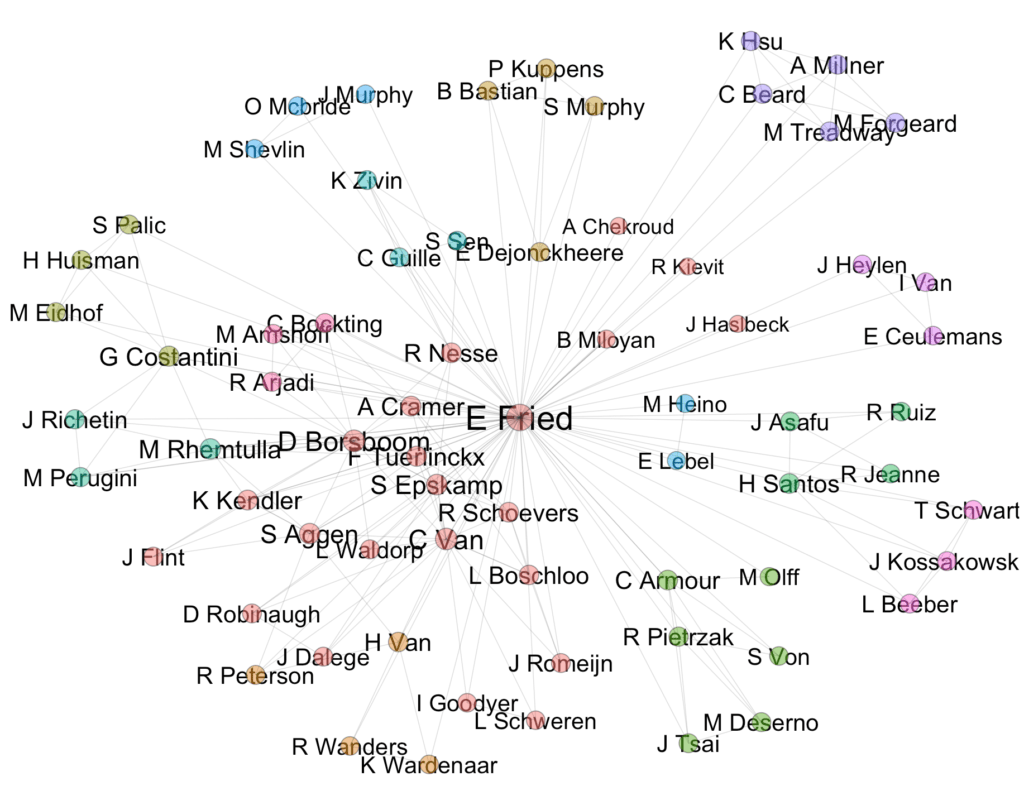# Create your collaborator network in R

I created a collaborator network last year in R, but based that on an excel input file that I created manually, and edited the names using Inkscape. Funny doing it once, but too much effort to repeat that every year.I have a larger number of collaborators now, so I looked into finding an automated way of getting the papers into a format from which I can plot the network. Unfortunately, I haven’t been too successful with the scholar() R package, and I decided to walk into the office 3 meters down the hall, where Sacha Epskamp waved his gsub() wand. The R code below is by no means a proper solution, and I’m largely sharing this to see whether others have found easy ways to export papers from a Google Scholar profile that contains all author names — not just the first 5 — into a reasonably clean database.

What we do to create my collaborator network is to export all citations in APA format into a text file; you can easily do that with your reference manager in a few seconds if you know what you’re doing. Here is the file to reproduce the analyses below.

In R, we load qgraph and dplyr, clean up the refs.txt file via a number of gsub() commands (all hail Sacha the gsub() king), and connect these via the dplyr %>% operator that allows us to put functions together without lots of nested parentheses.

```library("qgraph") library("dplyr")   # Load references: refs <- readLines("refs.txt")   authors <- refs %>% # First remove everything from date onwards: gsub("\\(.*","",.) %>% # Remove asterix: gsub("\\*","",.) %>% # Make lowercase: tolower %>% # Remove spaces: gsub("\\s*","",.) %>% # Remove & gsub("\\&","",.) %>% # Split on .,: strsplit(split="(?<=\\.),",perl=TRUE) %>% # Remove periods and everything following: lapply(function(x)gsub("\\..*","",x))```

You want to adapt this depending on the reference format you have your papers in.

Next up, we create a matrix where we have authors as rows and papers as columns. The numbers in this adjacency matrix indicate how often authors have been together on a given paper.

```# Collect unique authors: allAuthors <- unique(unlist(authors))   # Create list SymXDis <- do.call(cbind,lapply(authors,function(x){ 1*(allAuthors %in% x) }))   # Make adjacency: adj <- SymXDis %*% t(SymXDis)```

The last step is a trick that’ll save you a ton of time, multiplying the adjacency matrix by its inverse to obtain what we need to plot the network.

Finally, we define author names as last names, and then plot the network in different ways.

```# Labels: labs <- gsub(",.*","",allAuthors) substr(labs,0,1) <- toupper(substr(labs,0,1))   # Plot: sha <- ifelse(allAuthors == "fried,e","square", "circle") names<-c(1:71)   pdf("1network_full_numbers.pdf", width=6, height=4.5) qgraph(adj, labels = names, nodeNames = labs, color = "#eeeeee", shape=sha, vsize = 4, diag = FALSE, layout = "spring", edge.color = "#666666", repulsion = 0.90, border.width=2, border.color='#444444', label.color="#555555", legend.cex=.15) dev.off()   pdf("2network_full_names.pdf") qgraph(adj, labels = labs,color = "#eeeeee", vsize = 5, diag = FALSE, shape=sha, layout = "spring", edge.color = "#666666", repulsion = 0.90, border.width=2, border.color='#444444', label.color="#555555") dev.off()```

This gives us:We can also plot the networks excluding the focal node; this better highlights relationships among your co-authors (obviously, only for your papers, i.e. your co-authors might be unconnected although they published a paper together without you):

```# Without Eiko: adj2 <- adj[-1,-1]   pdf("3network_empty_numbers.pdf", width=6, height=4.5) qgraph(adj2, labels = names[-71], nodeNames = labs[-1], color = "#eeeeee", vsize = 4, diag = FALSE, layout = "spring", edge.color = "#666666", repulsion = 0.90, border.width=2, border.color='#444444', label.color="#555555", legend.cex=.15) dev.off()   pdf("4network_empty_names.pdf") qgraph(adj2, labels = labs[-1],color = "#eeeeee", vsize = 5, diag = FALSE, layout = "spring", edge.color = "#666666", repulsion = 0.90, border.width=2, border.color='#444444', label.color="#555555") dev.off()```To make this really pretty, you’d probably have to add a number of fixes (e.g. Dutch names such as van Loo are currently garbled into Vanloo), and identical last author names will eventually lead to issues.

If you have more elegant solutions based on crawling papers from Google Scholar, let us know in the comments.

Update February 2018:
The package scholarnetwork provides an easy way to do this now. However, some some additional cleaning work is required (similar to what we do above). Plotting the default network shows quite a few authors names that are incorrect.Code:

```# code from https://github.com/pablobarbera/scholarnetwork library(scholarnetwork) library(igraph)   d <- extractNetwork(id="DUK0qQoAAAAJ", n=500) str(d)   plotNetwork(d\$nodes, d\$edges, file="network.html")     library(ggplot2) # cleaning network data network <- graph_from_data_frame(d\$edges, directed=FALSE) set.seed(123) l <- layout.fruchterman.reingold(network, niter=1500) # layout fc <- walktrap.community(network) # community detection   # node locations nodes <- data.frame(l); names(nodes) <- c("x", "y") nodes\$cluster <- factor(fc\$membership) nodes\$label <- fc\$names nodes\$degree <- degree(network)   # edge locations edgelist <- get.edgelist(network, names=FALSE) edges <- data.frame(nodes[edgelist[,1],c("x", "y")], nodes[edgelist[,2],c("x", "y")]) names(edges) <- c("x1", "y1", "x2", "y2")   # and now visualizing it... p <- ggplot(nodes, aes(x=x, y=y, color=cluster, label=label, size=degree)) pq <- p + geom_text(color="black", aes(label=label, size=degree), show_guide=FALSE) + # nodes geom_point(color="grey20", aes(fill=cluster), shape=21, show_guide=FALSE, alpha=1/2) + # edges geom_segment( aes(x=x1, y=y1, xend=x2, yend=y2, label=NA), data=edges, size=0.25, color="grey20", alpha=1/5) + ## note that here I add a border to the points scale_fill_discrete(labels=labels) + scale_size_continuous(range = c(5, 8)) + theme( panel.background = element_rect(fill = "white"), plot.background = element_rect(fill="white"), axis.line = element_blank(), axis.text = element_blank(), axis.ticks = element_blank(), axis.title = element_blank(), panel.border = element_blank(), panel.grid.major = element_blank(), panel.grid.minor = element_blank(), legend.background = element_rect(colour = F, fill = "black"), legend.key = element_rect(fill = "black", colour = F), legend.title = element_text(color="white"), legend.text = element_text(color="white") ) + ## changing size of points in legend guides(fill = guide_legend(override.aes = list(size=5)))   pq```

## 5 thoughts on “Create your collaborator network in R”

1. Pingback: Caio Maximino

2.Palash Sharma

Hi Professor Fried,
It’s really a nice blog. I am kind of interested in social networking analysis and try to run your code. I am wondering when i try to run the “refs.txt” is gives a warning message and fails to plot the network graph. I am wondering what’s creates this problem. It would be so nice of you if you please respond me. Thanks in advance
Warning message:
In readLines(“refs.txt”) : incomplete final line found on ‘refs.txt’

1.Eiko Post author

Sorry for the late response Palash. I don’t know why reading in the txt file gives you trouble, it works on my machine. There are numerous ways to read in txt files, however, maybe just try a different one, or just the native one in R studio via the dropdown menu. Good luck!

This site uses Akismet to reduce spam. Learn how your comment data is processed.